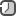### Factory design pattern in Java, Kotlin and Python

Creational Design Patterns provide solution to instantiate an object in the best possible way for specific situations. Following design patterns come under this category.

Factory pattern provides a way to delegate the instantiation logic to child classes.

Factory design pattern is used when we have a super class with multiple sub-classes and based on input, we need to return one of the sub-classes. This pattern takes out the responsibility of instantiation of a class from client program to the factory class.

Super class in factory pattern can be an interface or a normal Java class. In Python it is abstract class in most cases.

Benefits:

• Factory pattern provides approach to code for interface rather than implementation.
• Factory pattern removes the instantiation of actual implementation classes from client code, making it more robust, less coupled and easy to extend.
• Factory pattern provides abstraction between implementation and client classes through inheritance.

We're going to create a `Shape` interface and concrete classes implementing the `Shape` interface. A factory class `ShapeFactory` is defined as a next step.

Demo class will use `ShapeFactory` to get a `Shape` object. It will pass information (CIRCLE/ RECTANGLE/ SQUARE) to `ShapeFactory` to get the type of object it needs.

Now let's take a look at a sequence diagram to see how this pattern behaves:

Usage of Factory design pattern:

• When a class doesn't know what sub-classes will be required to create
• When a class wants that its sub-classes specify the objects to be created.
• When the parent classes choose the creation of objects to its sub-classes.

Java

Create an interface.

```# file Shape.java
public interface Shape {
void draw();
}
```

Create concrete classes implementing the same interface.

```# file Rectangle.java

public class Rectangle implements Shape {
@Override
public void draw() {
System.out.println("Inside Rectangle::draw() method.");
}
}

# file Square.java

public class Square implements Shape {
@Override
public void draw() {
System.out.println("Inside Square::draw() method.");
}
}

# file Circle.java

public class Circle implements Shape {
@Override
public void draw() {
System.out.println("Inside Circle::draw() method.");
}
}
```

Create a Factory to generate object of concrete class based on given information.

```# file ShapeFactory.java

public class ShapeFactory {
public Shape getShape(String shapeType){
if(shapeType == null){
return null;
}
if(shapeType.equalsIgnoreCase("CIRCLE")){
return new Circle();
} else if(shapeType.equalsIgnoreCase("RECTANGLE")){
return new Rectangle();
} else if(shapeType.equalsIgnoreCase("SQUARE")){
return new Square();
}
return null;
}
}
```

Use the Factory to get object of concrete class by passing an information such as type.

```# file FactoryPatternDemo.java

public class FactoryPatternDemo {
public static void main(String[] args) {
ShapeFactory shapeFactory = new ShapeFactory();
Shape shape1 = shapeFactory.getShape("CIRCLE");
shape1.draw();

Shape shape2 = shapeFactory.getShape("RECTANGLE");
shape2.draw();

Shape shape3 = shapeFactory.getShape("SQUARE");
shape3.draw();
}
}
```

Python 3

Create base class.

```import abc
class Shape(metaclass=abc.ABCMeta):
@abc.abstractmethod
def draw(self):
pass
```

Create concrete classes implementing the same base class.

```class Rectangle(Shape):
def draw(self):
print("Inside Rectangle::draw() method.")

class Square(Shape):
def draw(self):
print("Inside Square::draw() method.")

class Circle(Shape):
def draw(self):
print("Inside Circle::draw() method.")
```

Create a Factory to generate object of concrete class based on given information.

```class ShapeFactory(object):
@staticmethod
def get_shape(shape_type):
if shape_type == 'CIRCLE':
return Circle()
elif shape_type == 'RECTANGLE':
return Rectangle()
elif shape_type == 'SQUARE':
return Square()
return null
```

Use the Factory to get object of concrete class by passing an information such as type.

```if __name__ == '__main__':
rectangle = ShapeFactory.get_shape('RECTANGLE')
rectangle.draw()

circle = ShapeFactory.get_shape('CIRCLE')
circle.draw()

square = ShapeFactory.get_shape('SQUARE')
square.draw()
```

Kotlin

Create an interface.

```interface Shape {
fun draw()
}
```

Create concrete classes implementing the same interface.

```class Rectangle: Shape {
override fun draw() {
println("Inside Rectangle::draw() method.")
}
}

class Square: Shape {
override fun draw() {
println("Inside Square::draw() method.")
}
}

class Circle: Shape {
override fun draw() {
println("Inside Circle::draw() method.")
}
}
```

Create a Factory to generate object of concrete class based on given information.

```class ShapeFactory {
fun getShape(shapeType: String): Shape {
return when(shapeType.trim()) {
"CIRCLE" -> Circle()
"RECTANGLE" -> Rectangle()
"SQUARE" -> Square()
else -> throw RuntimeException("Unknown shape \$shapeType")
}
}
}
```

Use the Factory to get object of concrete class by passing an information such as type.

```fun main(args: Array<String>) {
val shapeFactory = ShapeFactory()

val shape1: Shape = shapeFactory.getShape("CIRCLE")
shape1.draw()

val shape2: Shape = shapeFactory.getShape("RECTANGLE")
shape2.draw()

val shape3: Shape = shapeFactory.getShape("SQUARE")
shape3.draw()
}
```